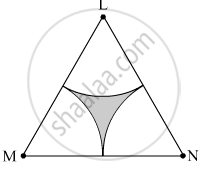# Δ Lmn is an Equilateral Triangle. Lm = 14 Cm. as Shown in the Figure, Three Sectors Are Drawn with Vertices as Centers and Radius 7 Cm. Find, Area of Any One of the Sector - Geometry

#### Question

$∆$ LMN is an equilateral triangle. LM = 14 cm. As shown in the figure, three sectors are drawn with vertices as centers and radius 7 cm.
Find, Area of any one of the sectors.#### Solution

∆LMN is an equilateral triangle.
∴ LM = MN = LN = 14 cm
∠L = ∠M = ∠N = 90º

Radius of the each sector, r = 7 cm
Area of any one of the sectors =$\frac{\theta}{360° } \times \pi r^2 = \frac{60° }{360° } \times \frac{22}{7} \times \left( 7 \right)^2$  = 25.67 cm2

Is there an error in this question or solution?